4. SPACE-MATTER-TIME UNITY AXIOM

We’d like to attract readers’ attention to the main reason of the theoretical physics crisis: lack of understanding of fundamental significance of the space-matter-time axiom. Its essence is in fact that separate existence of space, matter and time is impossible. It is impossible to separate matter from space and it is impossible to imagine their separate existence. It is impossible to separate time from space or from matter. In the reality where we exist space, matter and time are primary and inseparable elements of the universe. Axiomacity of  this statement is obvious , , .

The question is: haven’t mathematicians, physicists, chemists and other investigators of reality taken into account axiomacity of space-matter-time unity? The answer will be definite. No, they haven’t. Almost all modern physical theories contradict this axiomacity , , , , .

Space-matter-time unity axiom points out to the fact that correlation between matter, space and time should be reflected in all mathematical models which describe the changeable reality. But this simple rule (as it seems from the first sight) remained unnoticed neither by mathematicians, no physicists , .

For example, Lorents transformations serve as a basis fir  the special theory of relativity by A. Einstein. These transformations are derived from the main mathematical postulate on pseudo-Euclidean geometry by Minkovsky .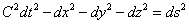.                                                      (1)

Let us pay attention to the fact that the equation (1) has another form in the Euclidean geometry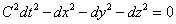.                                                        (2)

If we compare these two equations, we’ll see that the Euclidean geometry Cdt=OM is straight-line diagonal of the parallelepiped (Fig. 1), and in the equation (1) of Minkovsky’s geometry this diagonal cannot be straight-line, because this equation does not correspond to Pythagorean theorem. As value ds is available in Minkovsky’s equation (1), it makes the parallelepiped’s diagonal curvilinear OEM (Fig. 1), and this fact is undeniable.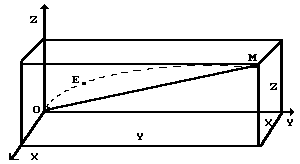Fig. 1 Diagram to the analysis of Minkovsky’s geometry

Let us pay attention to the fact that the length of the Cdt = OM diagonal is measured with the help of a photon, which moves the velocity C.

Straightness of the diagonal Cdt = OM on the equation (2) corresponds to the possibility of the photon to move in space straightway. Curvilinearity of the diagonal Cdt = OM  in Minkovsky’s equation (1) disagrees with its feature. It means that we have no right to put photon’s velosity C in the equation (1) postulated by Minkovsky, which is a basis of his four-dimensional geometry.

From the equation (2), we can determine the position of the photon on the diagonal Cdt = OM at any time. It corresponds to the axiom of space-matter-time unity. At every point of the diagonal Cdt= OM , the photon (matter), space and time exist as inseparable unity. Versa verse, Minkovsky’s equation (1) does not allow to determine the position of the photon on the track OEM at the present time violating space-matter-time unity. It results in incorrectness of the mathematical model (1), which is a basis of four-dimensional geometry of Minkovsky.

It is known that the fundamental postulates are worthy of note only when their reliability has been checked with the help of the direct experiments not once. The postulated mathematical model (1) of the pseudo-Euclidean geometry by Minkovsky is being used in science nearly 100 years without the check of its reliability with the help of a direct experiment. It results in incorrectness of many physical theories based on Minkovsky’s geometry. This incorrectness is easily checked with the help of the axiom of space-matter-time unity. Let us demonstrate it using the analysis of Lorent’s transformations as an example. These transformations are derived from the mathematical model (1)  of the pseudo-Euclidean geometry by Minkovsky , . Let us furnish them in a classical type: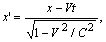(3)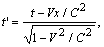(4)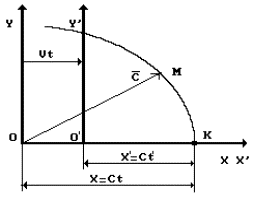Fig. 2. Diagram to analysis of Lorentz’s transformations

Let us pay attention to the fact that there is a coordinate x’ in the formula (3) which is registered in the mobile reference system (Fig. 2), and in the second formula only time t’ is present which passes in the same reference system. Thus, in the mathematical formulas (3) and (4) the change able value of space interval x’ in the mobile reference system is separated from time t’ passing in this reference system. As in reality it is impossible to separate space from time, the above-mentioned equations cannot be analyzed separately. It is a set of equations, and they should be analyzed together. Only this analysis will correspond to space-matter-time axiom, and the results of only this analysis will reflect reality. But this simple rule is still ignored by physics and mathematicians. It is clear from equation (3) that when V® C the space interval value x’ is reduced. They make a conclusion that if mobile system movement speed V is increased, the value of space interval x’ is reduced. Then they analyze only one equation (4). As follows from this equation, when V® C, value t’ is reduced.

Let us correct erroneous interpretation. As it is impossible to separate space from time in reality, let us analyze  equations (3) and (4) together; for this purpose let us divide the first one by the second one; we shall have the following result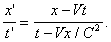(5)

The mathematical formula (5) reflects the dependence of coordinate x’ from t’. It means that formula (5) operates within the frames of space-matter-time axiom, i.e. within the frames of reality.

It is seen from Fig.2 that x is coordinate of light signal position in immovable reference system. It is equal to product of light movement speed C by time t. If we substitute equation x=Ct in the given formula (5), we shall get coordinate x’=Ct’ which registers light signal position in the mobile reference system. Where is this signal situated? As we change coordinates x and x’, it is situated on coinciding axes OX and OX’ at time moments t and t’, i.e. in point K (Fig. 2). Geometrical sense of Lorentz’s transformations is very simple. Coordinate x’ of point K in the mobile reference system and coordinate x in immovable reference system are registered in them (Fig. 2). It is a cross point of light sphere with axes OX and OX’.  This is the essence of the Lorentz transformations. There is no other information in these transformations, and they reflect no physical effects.

Later on, during the analysis of Dopler effect we’ll show that Lorentz transformations have played a role of a theoretical virus in theoretical physics of the 20th century.

This elementary analysis shows that Louis de Broglie’s wave equation, Schroedinger equation, Dirac equations well as all modern field physical theories contradict the space-matter-time unity axiom . It means that they describe physical world incompletely and cannot give us more information that they give now. That’s why the attempts to solve non-traditional problems with the help of these theories are unfruitful. Detailed information concerning these facts can be obtained from the  article , books , , , ,  and booklets , , .

This elementary analysis of Lorentz transformations shows that they conflict with the main axiom of natural science: the space-matter-time unity axiom. It appears from this they distort reality, that’s why they are erroneous.

It is natural that erroneousness of Lorentz transformations causes erroneousness of all theories based on the transformations. First of all, both theories of relativity of A. Einstein are erroneous , .

Availability of criterion for the estimation of the connection of any theory with reality allows us to estimate this connection of all theories, on which modern quantum physics is based.

Let us begin with Louis de Broglie’s monochrome wave equation.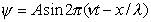(6)

In this equation l is the wavelength, v is wave frequency, x is the coordinate, and t is time. Let us take into consideration that in reality the movement of any object in space is synchronized with the course of time, i.e. coordinate x is always function of time t. In Louis de Broglie’s equation x and t are independent variables. It cannot happen in reality when coordinate x of the variable position of any object in space does not depend of time t. It means that Louis de Broglie’s equation conflicts with the main axiom of natural science: the space-matter-time unity axiom. That’s why we exclude it from our investigations.

Schroedinger’s equation in three-dimensional space looks more complicated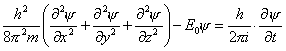(7)

The following function is the solution of this equation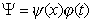,                                                                        (8)

In this function, coordinate x does not depend on time t. In this case, the solution result (8) conflicts with the space-matter-time unity axiom and is far from reality.

Nevertheless, Louis de Broglie’s equation and Schroedinger’s equation are widely used in quantum physics now; sometimes they describe the results of the experiments. As they are wave equations, it is natural that they can describe wave processes or the processes similar to them. The cause of independence of x from t in Louis de Broglie’s equation and in other equations is explained by the fact that  in wave geometry the variable function Y of oscillations at one and the same time can have the same value at various values of x. That’s why the results of the solutions of these equations have stochastic character and do not give the opportunity to find an exact value of any parameter. This result is caused by the fact that these equations are at variance with the space-matter-time unity axiom.

Sometimes the function (8) can be divided into two functions, each of them depends only on x or only on t, and it becomes possible to describe any process, which depends only on time t or on coordinate x. From function (8), it is possible to find function y=y(x), which gives the opportunity to calculate the range of hydrogen-type atoms.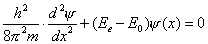(9)

It takes place due to the fact that the photon energy emitted by the electron during its energy jumps in the atoms depends only on distance x between the atomic nucleus and the electron in the moment of absorption or emission of the photon. But this result is an accidental one, because the classical equation has been found for the calculation of the spectra of not only hydrogen-type atoms, but of multi-electron atoms as well. We’ll give the deduction of this equation and show how it operates.

We are not going to give and analyse Dirac’s equation, because it has the same drawback as Louis de Broglie’s equation and Schroedinger’s equation. In this equation, coordinates x, y, z do not depend on time. That’s why it operates outside the framework of the space-matter-time unity axiom as well and does not give us the information, which could allow us to disclose electromagnetic structure of any particle .

The main role of axioms is to be a foundation of the new theories. The foundation of any future theory, which will be built on the grounds of the above mentioned axioms, will have everlasting strength.

We must mention the contradiction of differential equations in partial derivatives to the space-matter-time unity axiom. Usually, these equations include the time parameter; the change of other parameters is considered to be independent from time, and it conflicts with the space-matter-time unity axiom. Consequently, differential equations in partial derivatives reflect reality incompletely and can distort it sometimes. It has been found recently that there exists not one, but many solutions of Cauchy problem for the wave equation in partial derivatives .

As in the analysis of behaviour of elementary particles we are interested in actual, not stochastic character of this behaviour, we should try to find other equations, which differ from de Broglie’s equation, Schroedinger’s equation and Dirac’s equation.

Actually, quantum physics was born from the ratio, which describes energy of the photon , .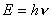,                                                                     (10)

That’s why it would be better to pay more attention to the analysis of this ratio in order to see if it conflicts with the classical physics laws actually.

First of all, it is necessary to define what law governs constancy of Planck’s constant h. It cannot be constant without a cause. Its constancy should be governed by one of the fundamental laws of Nature. And we’ll see that it is so indeed. The law of conservation of angular momentum, one of the fundamental laws of classical physics, governs constancy of Planck’s constant. That’s why the appearance of constant h in mathematical model of the black body radiation law has proved that fact that this law is a law of classical physics, not vice verse as it has taken place historically .

If we were lucky enough to disclose the structure of electromagnetic radiation of the black body, a classical interpretation of this radiation would take place, as a result. But it was not the case. The structure of the photon, a quantum of energy postulated by Max Planck, proved to be very complicated. That’s why we return to the analysis of experimental dependence of the black body radiation law and try to disclose the structure of the photon first of all. The law, which governs constancy of Planck’s constant and discloses classical interpretation of the black body radiation law, originates from this structure. Then we’ll give a classical deduction of mathematical model of the black body radiation law and see how all components of this law get a clear physical sense.

Next Page >>

The Foundations of Physchemistry of Microworld

Ó2003 Kanarev Ph. M.

Internet Version - http://book.physchemistry.innoplaza.net

<< Back to Physchemistry Book Index

 Spatial interval x’ is separated from time t’